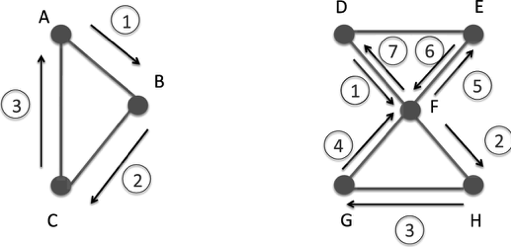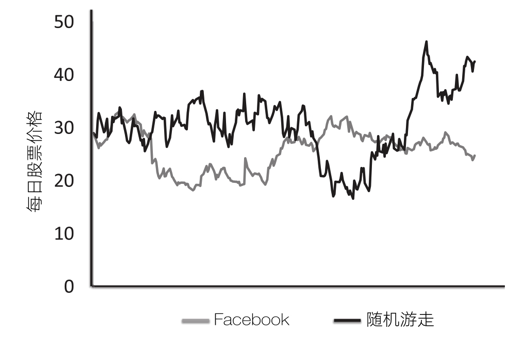## 伯努利瓮模型

$$N_G = N \times P$$

$$\sigma_{N_G} = \sqrt{N \times P \ times (1-P)}$$

## 随机走动模型

$$V_{t+1} = V_{t} + R(-1, 1)$$## 随机走动的应用